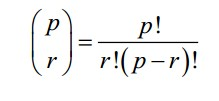# FuncTools in Python

In functional programming we use functions to produce the required output instead of objects. The primary difference between the two is that the state of objects changes continuously whereas functions have no state and make no changes to variables which Is not visible. The itertools functions is one of the most popular modules used to work with iterable collections. It includes functions that work with both finite and infinite iterators and the tee iterator which makes numerous copies of an iterator to be used independently.

The functools module in python is a very crucial library which helps create such functions, which are generally higher ordered functions since we modify existing functions to create new functions as required.

Functools or function tools help in customizing simple functions to create more complex functions by injecting another function.

Some of the most vital functions are decorators are:

• The LRU_cache decorator:
LRU stands for least recently used. A finite pool of recently used items are retained and the ones which are less frequently used are discarded. When we define the primitive Fibonacci recursive function, which calculates the nth Fibonacci number by recursively calculating the n-1th term and the n-2nd term, a lot of unnecessary calcluations are repeated. For example, to calculate f(50), we calculate f(49) and f(48). f(49) calculates f(48) again. Thus f(1) is calculated 98 times! If we store the value in the cache, a lot of calculations will end up getting saved. Without memoization, f(50) took 5946.281s to run and with memoization it took just 0.016s, a reduction of an unbelievable 3,71,625x.
• The reduce() function in the itertools module is a very powerful tool which can be used to create any number of higher ordered functions as per our convenience.
We can create the sum, count, min, max and sum of squares functions in the following manner using the reduce() function.
sum= lambda iterable: reduce(lambda x, y: x+y, iterable)
count= lambda iterable: reduce(lambda x, y: x+1, iterable, 0)
min= lambda iterable: reduce(lambda x, y: x if x < y else y, iterable)
max= lambda iterable: reduce(lambda x, y: x if x > y else y, iterable)
sum2= lambda iterable: reduce(lambda x,y: x+y**2, iterable, 0)
• The partial() function builds a new function from an existing function and a subset of the parameters. It binds the first parameter with a fixed value. For example,
Mod101=partial(fmod,101) takes only 1 parameter, the value of the modulo.
So Mod101(25) returns 101 % 25 = 1
• We can combine the reduce() and partial() functions to further simply these functions.
For example,
sum= partial(reduce, lambda x,y: x+y)
count= partial(reduce, lambda x,y: x+1)
sum2= partial(reduce, lambda x,y: x+y**2)
• We can combine map and reduce and utilise the power of functools to the fullest. Almost any function can be created using their combination.
def map_reduce(mapfunction, reducefunction, iterable):
return reduce(reducefunction, map(mapfunction, iterable))
This can be used as following:
def sumofsquares_using_mapandreduce(iterable):
return map_reduce(lambda y: y**2, lambda x,y: x+y, iterable)
• def count_using_mapandreduce(iterable):
return map_reduce(lambda y: 1, operator.add, iterable)

Take Away

Memoization is a powerful tool for optimization of recursive functions which call the function for the same values repeatedly. Another example of this is the calculation of the number of combinations of p different things taken r at a time. Each time, 3 different factorials are calculated. And each factorial recursively calls smaller factorials.There are many algorithms that can benefit from memoization of results. There are also some algorithms that might not benefit quite so much. Applications that work with flat values might not get optimized by memoization because floats differ by tiny values.

This random noise prevents the exact equality test in the lru cache decorator from working.

Interested in knowing more on such niche techniques? Check out http://research.busigence.com